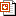# Laplace Approximation

Posted 2012.10.19 19:26
Laplace's method

In mathematicsLaplace's method, named after Pierre-Simon Laplace, is a technique used to approximate integrals of the form$\int_a^b\! e^{M f(x)} \, dx$

where ƒ(x) is some twice-differentiable functionM is a large number, and the integral endpoints aand b could possibly be infinite. This technique was originally presented in Laplace (1774, pp. 366–367).$\int_a^b\! e^{M f(x)}\, dx\approx \sqrt{\frac{2\pi}{M|f''(x_0)|}}e^{M f(x_0)} \mbox { as } M\to\infty. \,$

관련 논문과 발표 자료Laplace Approximation.pptx

정리한 것 SCAN#### 'Enginius > Machine Learning' 카테고리의 다른 글

 Solving LMI using SeDuMi  (0) 2012.11.26 2012.11.20 2012.10.19 2012.08.23 2012.07.26 2012.07.17